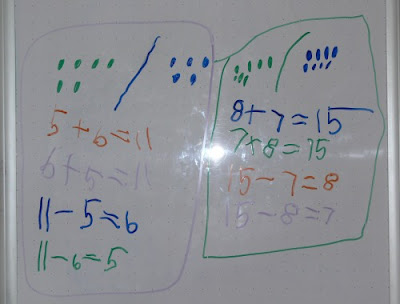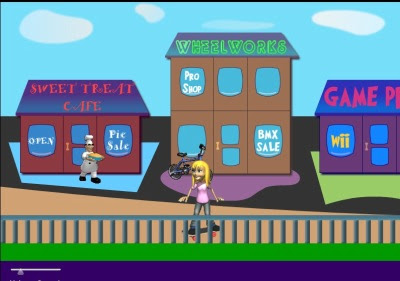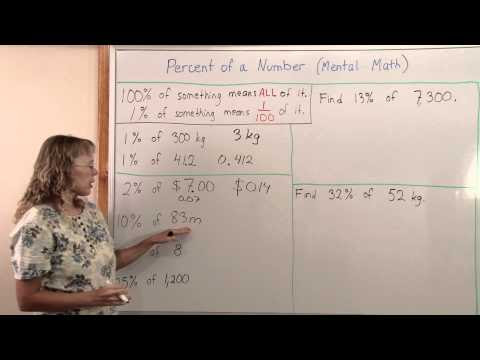## Posts

Showing posts from November, 2009

### Math Teachers at Play carnival

Denise has done a good job putting together Math Teachers at Play carnival #20 . Lots of stuff to read, head on over !

### Ratio word problem solved with block model and algebra

I guess it is time for some more problem solving, since someone sent this question in. Two numbers are in the ratio of 1:2. If 7 be added to both, their ratio changes to 3:5. What is the greater number? We can model the two original numbers with blocks. 1 block and 2 blocks makes the ratio to be 1:2. |-------| |-------|-------| Now add the same thing to both (the 7): 7 |-------|---| |-------|-------|---| 7 The way I just happened to draw these suggests that I could just split the original block in two, and the problem is solved: 7 |---|---|---| |---|---|---|---|---| 7 Here, each little block is 7. The original larger blocks are 14 each. So the original bigger number, which had two larger blocks, is 28, and the smaller number is 14. Check: Their ratio is 28:14 = 2:1. If you add 7 to both, you have 35 and 21, and their ratio is 35:21 = 5:3. Solving the same problem using algebra The two numbers in the ratio of 1:2 are

### Mixture problems - algebra 1

I am hoping you can help me. I can not remember how to solve mixture problems and how to set them up. Examples are as follows: A merchant made a mixture of 150lb. of tea worth \$109.50 by mixing tea worth \$1.25 a pound with tea worth \$.65 a pound. How many pounds of each kind did he use? Organizing the information in a table or chart is usually very helpful in dealing with mixture problems. Other than that, it helps to study several examples and practice solving them yourself. After a while, it gets easier and patterns begin to emerge. The first problem has two unknowns. Let x be the amount of more expensive tea, and y the amount of the cheaper tea (in pounds). In our table, we will look at the amounts of tea (in pounds), price per pound, AND the amount the tea is worth, which is (the amount) times (the price). amount | price per lb | worth ------------------------------------- x | \$1.25 | 1.25x ------------------------------------- y | \$0.65

### Fact families on a whiteboardI just found this picture that I took of the fact families my 4-year old wrote on the whiteboard - totally on her own. There was a time she loved writing fact families like this every day. Being able to choose different color markers plus it being on the whiteboard seemed to be the main motivating factors, because she didn't want to write them on blank paper... Kids are funny. Then again, it allows us teachers to use colorful markers as a "motivational tool" : ) Anyway, I was really happy that she had grasped the concept.

### Review of Math ApprenticeMath Apprentice is a new free website, meant to show students how math is used in real world. In the game, you are like an apprentice at various companies, applying your math skills to challenges similar to those encountered in the real world and real companies. Main Street - Click to enlarge To begin, you click the button on the home page of the site that says "Explore the Math". Then choose your character, and you'll be on the main street (see screenshot above) . Then use arrow keys to move right or left, and click to select a company to visit. The companies you can visit are: Sweet Treat Cafe - baking pies Sweet Treat Cafe - Click to enlarge Wheelworks - constructing bicycles and exploring gear ratios Game Pro! - keep track of the distance between superhero and the villain in a computer game, using Pythagorean Theorem Spacelogic - study speed of a spacecraft & slope, and then angle & distance commands to get the space rover where it needs go. Trig

### Percentages with mental math(This is an older post that I have revised plus added a video to it. ) In this article I want to explore some ideas for using MENTAL math in calculating percent s or percentages. I have also made a video about this topic: And here are the ideas: Find 10% of some example numbers (by dividing by 10). Find 1% of some example numbers (by dividing by 100). Find 20%, 30%, 40% etc. of these numbers. FIRST find 10% of the number, then multiply by 2, 3, 4, etc. For example, find 20% of 18. Find 40% of \$44. Find 80% of 120. I know you can teach the student to go 0.2 × 18, 0.4 × 0.44, and 0.8 × 120 - however when using mental math , the above method seems to me to be more natural. Find 3%, 4%, 6% etc. of these numbers. FIRST find 1% of the number, then multiply. Find 15% of some numbers. First find 10%, halve that to find 5%, and add the two results. Find 25% and then 75% of some numbers. 25% of a number is 1/4 of it, so you find it by dividing by 4. For example, 25% of 16 is 4.